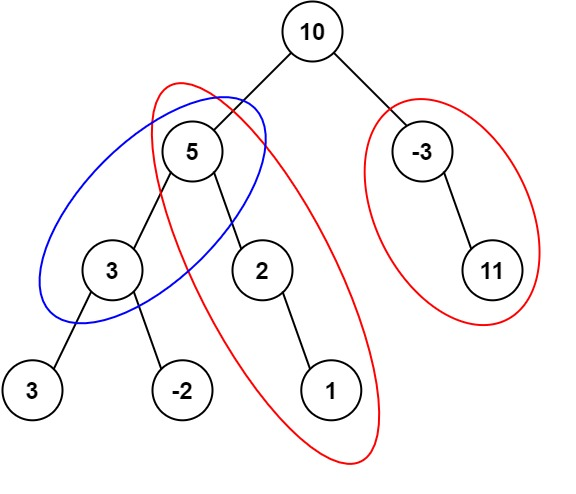# GeetCode Hub

Given the `root` of a binary tree and an integer `targetSum`, return the number of paths where the sum of the values along the path equals `targetSum`.

The path does not need to start or end at the root or a leaf, but it must go downwards (i.e., traveling only from parent nodes to child nodes).

Example 1:```Input: root = [10,5,-3,3,2,null,11,3,-2,null,1], targetSum = 8
Output: 3
Explanation: The paths that sum to 8 are shown.
```

Example 2:

```Input: root = [5,4,8,11,null,13,4,7,2,null,null,5,1], targetSum = 22
Output: 3
```

Constraints:

• The number of nodes in the tree is in the range `[0, 1000]`.
• `-109 <= Node.val <= 109`
• `-1000 <= targetSum <= 1000`

/** * Definition for a binary tree node. * public class TreeNode { * int val; * TreeNode left; * TreeNode right; * TreeNode() {} * TreeNode(int val) { this.val = val; } * TreeNode(int val, TreeNode left, TreeNode right) { * this.val = val; * this.left = left; * this.right = right; * } * } */ class Solution { public int pathSum(TreeNode root, int targetSum) { } }